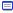ラシウラ出張所2008/06/16

■ [Haskell]λ式のde Bruijn Index化と評価data Term = Var Int
| App Term Term
| Lam Term
| Con String
deriving Show

eval :: Term -> Term
eval (Var n) = Var n
eval (Con s) = Con s
eval (Lam b) = Lam b
eval (App (Lam b) opd) =
eval (replace 0 b (eval opd))
eval (App opr opd) =
eval (App (eval opr) opd)

replace :: Int -> Term -> Term -> Term
replace var (Lam b) arg = Lam (replace (var+1) b arg)
replace var (App opr opd) arg =
App (replace var opr arg) (replace var opd arg)
replace var (Con s) arg = Con s
replace var (Var n) arg | n < var = Var n
replace var (Var n) arg | n == var = fixfree 0 var arg
replace var (Var n) arg | n > var = Var (n-1)

fixfree :: Int -> Int -> Term -> Term
fixfree depth var (Lam b) = Lam (fixfree (depth+1) var b)
fixfree depth var (App opr opd) =
App (fixfree depth var opr) (fixfree depth var opd)
fixfree depth var (Con s) = Con s
fixfree depth var (Var n) | n <= depth = Var n
fixfree depth var (Var n) | n > depth = Var (n+var-1)

-- examples
icomb = Lam (Var 0)
kcomb = Lam (Lam (Var 1))
scomb = Lam (Lam (Lam (App (App (Var 2) (Var 0)) (App (Var 1) (Var 0)))))
bcomb = Lam (Lam (Lam (App (Var 2) (App (Var 1) (Var 0)))))
ccomb = Lam (Lam (Lam (App (App (Var 2) (Var 0)) (Var 1))))
ycomb = Lam (App (Lam (App (Var 1) (App (Var 0) (Var 0))))
(Lam (App (Var 1) (App (Var 0) (Var 0)))))

-- eval (App (App (App scomb kcomb) kcomb) (Con "a")) => Con "a"

data Expr = V String
| A Expr Expr
| L String Expr
| C String
deriving Show

compile :: [String] -> Expr -> Term
compile namestack (C s) = Con s
compile namestack (A opr opd) =
App (compile namestack opr) (compile namestack opd)
compile namestack (L p b) = Lam (compile (p:namestack) b)
compile namestack (V v) = Var (find 0 v namestack)
where
find index var (name:rest) = if var == name then index
else find (index+1) var rest

-- compile [] (L "x" (L "y" (V "x"))) => Lam (Lam (Var 1))
-- compile [] (L "f" (A (L "x" (A (V "f") (A (V "x") (V "x")))) (L "x" (A (V "f") (A (V "x") (V "x"))))))